U.S. Department of Transportation
1200 New Jersey Avenue, SE
Washington, DC 20590
202-366-4000

Federal Highway Administration Research and Technology
Coordinating, Developing, and Delivering Highway Transportation InnovationsThis report is an archived publication and may contain dated technical, contact, and link information
 Federal Highway Administration > Publications > Research Publications > 06139 > Appendb.Cfm Traffic Detector Handbook:Third Edition—Volume II
 Publication Number: FHWA-HRT-06-139 Date: October 2006

# Traffic Detector Handbook:Third Edition—Volume II

## APPENDIX B.CURRENT SHEET FORMULA FOR CALCULATION OF LOOP INDUCTANCE

A formula for calculating the inductance produced by a rectangular current sheet is given by(1)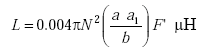(B-1)

where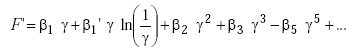(B-2)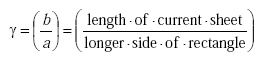(B-3)
 N = number of turns and α1 = length of shorter side of loop.
 Inductive loops are modeled as very short solenoids.

The term, “length of current sheet,” is defined as the axial length of a coil or solenoid. For an inductive loop, the length of the current sheet is equivalent to the height of the wires in the slot, as described in the sample calculation that follows. Since the length of the current sheet is very small compared to the longer side of the rectangle, γ is also very small. The factor F’ adjusts for the fact that inductive loops perform as very short solenoids (i.e., the loop area is much greater than the turn spacing).

The values of β are obtained from Table B-1.  The factor K that appears in the table is given by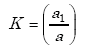(B-4)
Table B-1. Values of β coefficients for short rectangular solenoids.(2)
Kβ1β1β2β3β5β7
1.00 0.46220.63660.2122−0.00460.0046−0.0382
0.95 0.45740.65340.2234−0.00460.0053
0.90 0.45120.67200.2358−0.00460.0064−0.0525
0.85 0.44480.69280.2496−0.00420.0080
0.80 0.43640.71620.2653−0.00310.0103−0.0831
0.75 0.42600.74270.2829−0.00100.0141
0.70 0.41320.77300.30320.00260.0198−0.1564
0.65 0.39710.80800.32650.00850.0291
0.60 0.37670.84880.35370.01790.0432−0.3372
0.55 0.35000.89700.38580.03310.0711
0.50 0.31510.95490.42440.05780.1183−0.7855
0.40 0.18361.11410.53050.16970.3898−2.403
0.30 0.03141.33590.70740.54332.0517−7.85
0.20 −0.64091.90991.06102.323014.50715.51
0.10 −3.23093.50142.122022.5480497.3614280.00

### SAMPLE INDUCTANCE CALCULATION

An example from reference 1 is used to demonstrate the calculation of the self inductance for a short, three-turn, 6- by 6-foot (1.8- by 1.8-m) loop, using the current sheet model described above.

 The following parameters are used:
 a1 = 6 ft = 182.88 cm a = 6 ft = 182.88 cm N = 3 turns P = turn spacing = 150 mils = 0.381 cm b = N P = (3)(0.381) = 1.143 cm γ  = (b/a) = 1.143/182.88 = 0.00625 cm K = (a1 / a) = 1.

From Table B-1,

 β1 = 0.4622 β1’ = 0.6366 β2 = 0.2122 β3 = -0.0046 β5 = 0.0046 β7 = -0.0382.

Solving Equation B-2 for F’ yields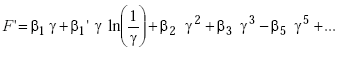(B-5)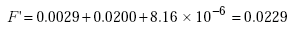(B-6)

where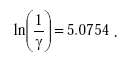(B-7)

Using Equation B-1, the inductance of the loop is found as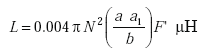(B-8)

or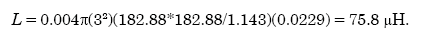(B-9)

### REFERENCES

1. Grover, F.W. Inductance Calculations. Dover Publications, Inc. 1962.
2. Y. Niwa, “A Study of Coils Wound on Rectangular Frames with Special Reference to the Calculation of Inductance,” Research of the Electrotechnical Laboratory, No. 141, Tokyo, Japan, 1924.